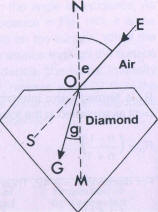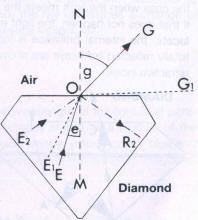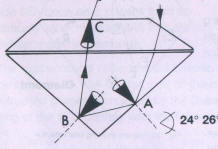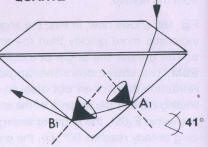## Internal brilliance

The incident light which passes through the surface of a stone is refracted. In principle, refraction takes place towards the normal when a ray of light passes from a thin to an optically denser medium. When the ray passes to a medium of lower optical density, the refraction is away from the normal. The greater the difference in density between the two media, the stronger the deflection of the light from its original direction of propagation.

The optical density is characterized by the velocity of light in these media. For example, in air a ray of light travels at a speed of C1 = 300,000 km/sec, but the speed in diamond is C2 = 124,120 km/sec, The ratio of the velocity of light in air and in a denser medium shows the refractive index of the medium.

n Diamond = 300000 km/sec = 2.417 = 2.42
124120 km/secDiamond is therefore the medium of greater density; a ray of light passing from air into a diamond will be bent towards the normal. To clarify the process of refraction it is again illustrated in Fig, 185.

A ray of light E° falls onto the table of a diamond at point O. A small part of the light is reflected by the surface, the larger part enters the stone, Once inside the diamond, the light ray E° does not continue along path as, but is refracted towards the normal along the direction OG. because it passes from an optically rarer medium (air) into the denser material (diamond).

The mathematical relationship between the light velocities C1 and C2 in adjacent media as well as the sine of the angle of incidence e and the angle of refraction g were formulated by the Dutch scientist Snell as early as the beginning of the 17th century with the following equation:

C1 = sin e = n = light refraction
C2   sin g

The ratio of the sin value of both angles or the ratio of the velocities of light to one another is a constant and is termed "n" for light refraction.

From this relationship the angle of refraction g is calculated when a light ray passes from air into diamond:

sin g = sin e X (c1 / c2 )

sin g = sin e X (124,120 / 300,000) = sin e X 0.4137

It is easily seen that light, when falling perpendicularly (e = 0), because sin 0° = 0: is not refracted. At an angle of incidence of 10° the angle of refraction g in diamond is 5° (sin 10° = 0.1738), at an angle of incidence of 20° (sin 20° = 0.342) It is about 9°, and so on. Strictly speaking the refractive index n alters with the wave-length of the incident light. As white visible light comprises a certain spread of wave-lengths, the refraction of gemstones is generally referred to the Invariable and reproducible wave-length of the so-called sodium line at 589.3 nm. The influence of wave-length on the refraction will be more fully explained In connection with dispersion.

A knowledge of the laws of refraction is indispensable for understanding total reflection, explained below; this is what evokes the internal brilliance of cut diamonds.The above law of refraction (Snell's Law) is universally valid; it is also applicable to rays which are emerging from the diamond in the reverse direction. In this case the rays are refracted away from the normal.

Fig 186 shows how, in this, the angle g increases more quickly than the angle e. The greater the angle of incidence EOM, the wider does the angle of ,refraction NOG open out also. In the limiting case, if the angle e in the stone lies within E1OM, the angle of emergence g already reaches 90°, i.e. the emerged ray travels along the stone's surface. If the angle e increases beyond E10, the ray is totally reflected within the dense medium. In Fig 188 this condition is illustrated by the ray E2O which in accordance with the law of reflection, is reflected in the direction OR2.

The reflection without loss of light within the dense medium is called total reflection. The ray E1O, whose refracted ray in the air reaches 90° to the normal, is the limiting ray of total reflection, and encloses the critical angle E1OM.
To work out the critical angle, using' Snell's equation:

sin e = sin g X 1/n

when angle g = 90° and sin 90° = 1, this immediately gives:

Since n = 2.42 (for air/diamond), then the critical angle e =

e = 24°26'

Thus the critical angle is entirely dependent on the refractive index; the larger the refractive index n, the smaller is the critical angle.

In cut diamonds total internal reflection plays a decisive role. The pavilion facets are responsible for the correct path of light in the stone. They must consequently be so arranged that all possible light which is incident through the crown of a brilliant, is totally reflected and directed upwards. That is only the case when the light meets the pavilion facets outside the critical angle. If that does not happen, the light emerges from the stone through the pavilion facets; the internal brilliance is lost. In Figs. 187 a and 187 b the paths of totally. reflected light-rays are shown, as exemplified in two stones of different refractive index.

 DIAMOND QUARTZFig 187 a Light refraction and total reflection in diamond Fig 187 b Light refraction and total reflection in quartz

The critical angle in Figs. 187 a and b is shown by a cone; by this means the critical angle surrounding the incidence normal on the pavilion facets can be envisaged.

A ray of light enters through the crown of a diamond brilliant and through that of a quartz brilliant of similar proportions, and in both stones meets the pavilion facets (A in diamond, A1 in quartz) outside the critical angle, and is then totally reflected to B and B1, In the rock crystal it meets B1, within the critical angle which in quartz is considerably greater than in diamond (41°). The ray of light therefore does not undergo total reflection, but is straight away refracted by the pavilion facets of the quartz into the air. In the diamond brilliant, with its small critical angle (24°26'), on the other hand, the ray of light meets B outside the critical angle and undergoes a second total reflection. It is reflected upwards and finally at C meets the crown facets. There, to be sure, in order to be able to emerge and be visible to the observer as internal brilliance, it must emerge within the critical angle.

As the light emerges from the stone's interior, then dispersion comes into play, which will be dealt with in the following section.

 External brilliance Dispersive brilliance